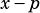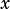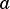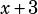A question on polynomials practice question #10 (p. 149 in PWN the SAT Math Guide): I understand how to solve using polynomial long division but can you explain your shortcut from the answer explanation? How would we know to plug in -3?

Sure, here’s the question:

The shortcut in the solution is to plug in, and that comes from the bolded text on page 145 of the book. If you want to know the remainder when a polynomial is divided by, whereis some constant, then pluginto the polynomial forand see what comes out. If you get 0, thenis a factor of the polynomial. If you get something other than 0, it’s the remainder.

Since this question is just asking for, the remainder, we can just follow that shortcut. Dividing byis the same as dividing by, which is why we plug in. Does that help?

There’s more on this concept on pages 144 and 145 of the book.Jan says: